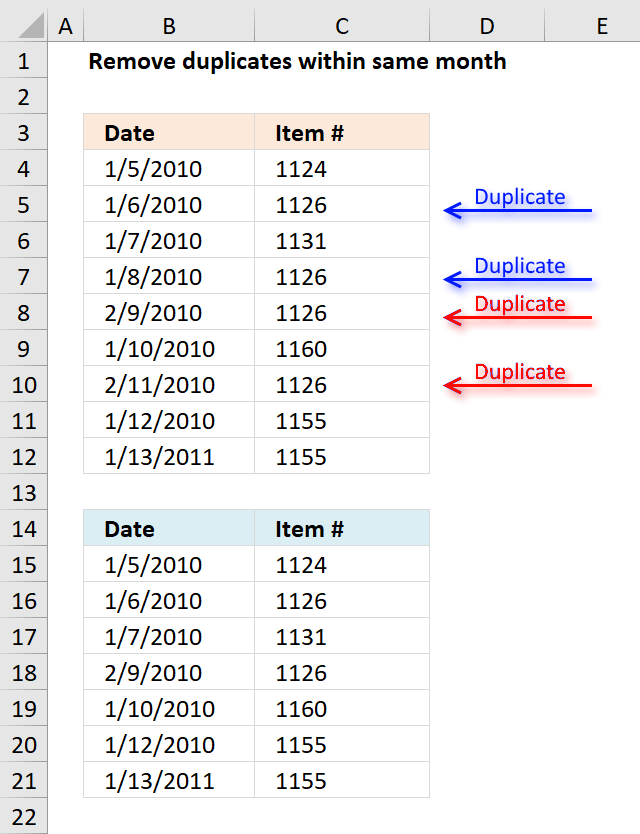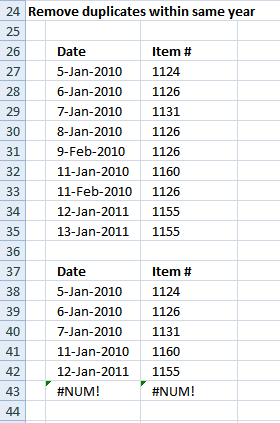Author: Oscar Cronquist Article last updated on December 19, 2018The array formula in cell B15 extracts dates from B4:B12 if it is not a duplicate item in the same month and year.

Array formula in B15:

=INDEX(\$B\$4:\$B\$12, SMALL(IF(MATCH(YEAR(\$B\$4:\$B\$12)&"-"&MONTH(\$B\$4:\$B\$12)&"-"&\$C\$4:\$C\$12, YEAR(\$B\$4:\$B\$12)&"-"&MONTH(\$B\$4:\$B\$12)&"-"&\$C\$4:\$C\$12, 0)=MATCH(ROW(\$B\$4:\$B\$12), ROW(\$B\$4:\$B\$12)), MATCH(ROW(\$B\$4:\$B\$12), ROW(\$B\$4:\$B\$12)), ""), ROWS(\$A\$1:A1)))

To enter an array formula, type the formula in a cell then press and hold CTRL + SHIFT simultaneously, now press Enter once. Release all keys.

The formula bar now shows the formula with a beginning and ending curly bracket telling you that you entered the formula successfully. Don't enter the curly brackets yourself.

Array formula in C15:

=INDEX(\$C\$4:\$C\$12, SMALL(IF(MATCH(YEAR(\$B\$4:\$B\$12)&"-"&MONTH(\$B\$4:\$B\$12)&"-"&\$C\$4:\$C\$12, YEAR(\$B\$4:\$B\$12)&"-"&MONTH(\$B\$4:\$B\$12)&"-"&\$C\$4:\$C\$12, 0)=MATCH(ROW(\$B\$4:\$B\$12), ROW(\$B\$4:\$B\$12)), MATCH(ROW(\$B\$4:\$B\$12), ROW(\$B\$4:\$B\$12)), ""), ROWS(\$A\$1:A1)))

### Explaining formula in cell B15

#### Step 1 - Concatenate year, month and item value

The YEAR function returns the year of an Excel date, the MONTH function returns the MONTH of an Excel date.

YEAR(\$B\$4:\$B\$12)&"-"&MONTH(\$B\$4:\$B\$12)&"-"&\$C\$4:\$C\$12

becomes

YEAR({40183; 40184; 40185; 40186; 40218; 40188; 40220; 40190; 40556})&"-"&MONTH({40183; 40184; 40185; 40186; 40218; 40188; 40220; 40190; 40556})&"-"&{1124; 1126; 1131; 1126; 1126; 1160; 1126; 1155; 1155}

The & ampersand sign lets you concatenate values, in this case, row-wise.

YEAR({40183; 40184; 40185; 40186; 40218; 40188; 40220; 40190; 40556})&"-"&MONTH({40183; 40184; 40185; 40186; 40218; 40188; 40220; 40190; 40556})&"-"&{1124; 1126; 1131; 1126; 1126; 1160; 1126; 1155; 1155}

returns

{"2010-1-1124"; "2010-1-1126"; "2010-1-1131"; "2010-1-1126"; "2010-2-1126"; "2010-1-1160"; "2010-2-1126"; "2010-1-1155"; "2011-1-1155"}

#### Step 2 - Match concatenated values to identify duplicates

The MATCH function finds the relative position of a value in an array or cell range.

MATCH(YEAR(\$B\$4:\$B\$12)&"-"& MONTH(\$B\$4:\$B\$12)&"-"&\$C\$4:\$C\$12, YEAR(\$B\$4:\$B\$12)& "-"&MONTH(\$B\$4:\$B\$12)& "-"&\$C\$4:\$C\$12, 0)

becomes

MATCH{"2010-1-1124"; "2010-1-1126"; "2010-1-1131"; "2010-1-1126"; "2010-2-1126"; "2010-1-1160"; "2010-2-1126"; "2010-1-1155"; "2011-1-1155"}, {"2010-1-1124"; "2010-1-1126"; "2010-1-1131"; "2010-1-1126"; "2010-2-1126"; "2010-1-1160"; "2010-2-1126"; "2010-1-1155"; "2011-1-1155"}, 0)

and returns

{1; 2; 3; 2; 5; 6; 5; 8; 9}

#### Step 3 - Compare value to sequence

If number is equal to corresponding number in sequence the logical expression returns TRUE.

MATCH(YEAR(\$B\$4:\$B\$12)&"-"& MONTH(\$B\$4:\$B\$12)&"-"& \$C\$4:\$C\$12,YEAR(\$B\$4:\$B\$12)&"-"& MONTH(\$B\$4:\$B\$12)&"-"& \$C\$4:\$C\$12,0)=MATCH(ROW(\$B\$4:\$B\$12), ROW(\$B\$4:\$B\$12))

becomes

{1; 2; 3; 2; 5; 6; 5; 8; 9}=MATCH(ROW(\$B\$4:\$B\$12), ROW(\$B\$4:\$B\$12))

becomes

{1; 2; 3; 2; 5; 6; 5; 8; 9}={1;2;3;4;5;6;7;8;9}

and returns

{TRUE; TRUE; TRUE; FALSE; TRUE; TRUE; FALSE; TRUE; TRUE}

#### Step 4 - Replace TRUE with corresponding row number

The IF function has three arguments, the first one must be a logical expression. If the expression evaluates to TRUE then one thing happens (argument 2) and if FALSE another thing happens (argument 3).

IF(MATCH(YEAR(\$B\$4:\$B\$12)&"-"&MONTH(\$B\$4:\$B\$12)&"-"&\$C\$4:\$C\$12, YEAR(\$B\$4:\$B\$12)&"-"&MONTH(\$B\$4:\$B\$12)&"-"&\$C\$4:\$C\$12, 0)=MATCH(ROW(\$B\$4:\$B\$12), ROW(\$B\$4:\$B\$12)), MATCH(ROW(\$B\$4:\$B\$12), ROW(\$B\$4:\$B\$12)), "")

becomes

IF({TRUE; TRUE; TRUE; FALSE; TRUE; TRUE; FALSE; TRUE; TRUE}=MATCH(ROW(\$B\$4:\$B\$12), ROW(\$B\$4:\$B\$12)), MATCH(ROW(\$B\$4:\$B\$12), ROW(\$B\$4:\$B\$12)), "")

becomes

IF({TRUE; TRUE; TRUE; FALSE; TRUE; TRUE; FALSE; TRUE; TRUE}, {1;2;3;4;5;6;7;8;9}, "")

and returns

{1;2;3;"";5;6;"";8;9}.

#### Step 5 - Extract k-th smallest row number

To be able to return a new value in a cell each I use the SMALL function to filter column numbers from smallest to largest.

The ROWS function keeps track of the numbers based on an expanding cell reference. It will expand as the formula is copied to the cells below.

SMALL(IF(MATCH(YEAR(\$B\$4:\$B\$12)&"-"&MONTH(\$B\$4:\$B\$12)&"-"&\$C\$4:\$C\$12, YEAR(\$B\$4:\$B\$12)&"-"&MONTH(\$B\$4:\$B\$12)&"-"&\$C\$4:\$C\$12, 0)=MATCH(ROW(\$B\$4:\$B\$12), ROW(\$B\$4:\$B\$12)), MATCH(ROW(\$B\$4:\$B\$12), ROW(\$B\$4:\$B\$12)), ""), ROWS(\$A\$1:A1))

becomes

SMALL({1;2;3;"";5;6;"";8;9}, ROWS(\$A\$1:A1))

becomes

SMALL({1;2;3;"";5;6;"";8;9}, 1)

and returns 1.

#### Step 6 - Get value

The INDEX function returns a value based on a cell reference and column/row numbers.

INDEX(\$C\$4:\$C\$12, SMALL(IF(MATCH(YEAR(\$B\$4:\$B\$12)&"-"&MONTH(\$B\$4:\$B\$12)&"-"&\$C\$4:\$C\$12, YEAR(\$B\$4:\$B\$12)&"-"&MONTH(\$B\$4:\$B\$12)&"-"&\$C\$4:\$C\$12, 0)=MATCH(ROW(\$B\$4:\$B\$12), ROW(\$B\$4:\$B\$12)), MATCH(ROW(\$B\$4:\$B\$12), ROW(\$B\$4:\$B\$12)), ""), ROWS(\$A\$1:A1)))

becomes

INDEX(\$C\$4:\$C\$12, 1)

and returns 1/5/2010 in cell B15.

Remove-duplicates-in-same-month.xlsx

### Remove duplicates within same year in excelArray formula in B38:

=INDEX(\$B\$27:\$B\$35, SMALL(IF(MATCH(YEAR(\$B\$27:\$B\$35)&"-"&\$C\$27:\$C\$35, YEAR(\$B\$27:\$B\$35)&"-"&\$C\$27:\$C\$35, 0)=ROW(\$B\$27:\$B\$35)-MIN(ROW(\$B\$27:\$B\$35))+1, ROW(\$B\$27:\$B\$35)-MIN(ROW(\$B\$27:\$B\$35))+1, ""), ROW(A1)))

Array formula in C38:

=INDEX(\$C\$27:\$C\$35, SMALL(IF(MATCH(YEAR(\$B\$27:\$B\$35)&"-"&\$C\$27:\$C\$35, YEAR(\$B\$27:\$B\$35)&"-"&\$C\$27:\$C\$35, 0)=ROW(\$B\$27:\$B\$35)-MIN(ROW(\$B\$27:\$B\$35))+1, ROW(\$B\$27:\$B\$35)-MIN(ROW(\$B\$27:\$B\$35))+1, ""), ROW(A1)))